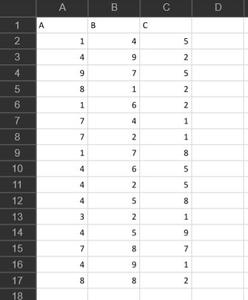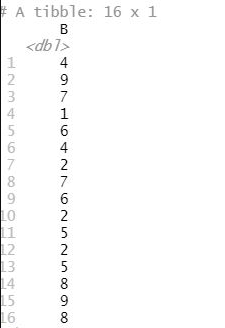# How to convert Excel column to vector in R ?

• Last Updated : 17 Jun, 2021

In this article, we will be looking at the different approaches to convert the Excel columns to vector in R Programming language.

The approaches to convert Excel column to vector in the R language are listed as follows:

1. Using \$-Operator with the column name.
2. Using the method of Subsetting column.
3. Using  pull function from dplyr library from the R language

Method 1: Using \$-Operator with the column name

In this method, we will be simply using the \$-operator with the column name and the name of the data read from the Excel file. Here, the name of the column name which has to be converted in the vector would be written at the end of the \$-operator and the name of the data read from the Excel file will be written before the \$-operator.

Syntax: dataframe\$column

Example:

In this example, we will be using the \$-Operator with the column name to convert the first column of the excel file in the form of a vector.

The used Excel file:Below is the implementation:

## R

 `library``(readxl)``gfg_data <- ``read_excel``(``"R/Data_gfg.xlsx"``)` `# \$-Operator``v1<-gfg_data\$A                        ``print``(v1)`

Output:

` 1 4 9 8 1 7 7 1 4 4 4 3 4 7 4 8`

Method 2: Using the method of Subsetting column

Under this approach user just need to enter the column name inside the brackets columns with the data to convert that excel column into vector form. This will only be converting the column to the vector which the user has passed with the brackets along with its data.

Syntax: dataframe[row_name,column_name]

Example:

In this example, we will be using the method of Subsetting column name to convert the second column of the Excel file in the form of a vector.

Below is the implementation:

## R

 `library``(readxl)``gfg_data <- ``read_excel``(``"R/Data_gfg.xlsx"``)` `# Subsetting column``v2 ＜- gfg_data[ , ``"B"``]                  ``print``(v2)`

Output:Method 3: Using pull function from dplyr library from the R language:

Here, the user has to call the pull() function from the dplyr library of R language and pass the column name which is needed to be converted into the vector form and the name of the datafile read variable.

pull function: This function pull selects a column in a data frame and transforms it into a vector.

Syntax: pull(.data, j)

Parameters:

• .data:-name of the data
• j:-The column to be extracted.

Returns: A vector of the provided column.

Example:

In this example, we will be using the method of Subsetting column name to convert the last column of the Excel file in the form of a vector.

Below is the implementation:

## R

 `library``(readxl)``gfg_data <- ``read_excel``(``"R/Data_gfg.xlsx"``)` `# Load dplyr``library``(``"dplyr"``)                      ` `# pull function``v3 ＜- ``pull``(gfg_data,C)                 ``print``(v3)`

Output:

` 5 2 5 2 2 1 1 8 5 5 8 1 9 7 1 2`

My Personal Notes arrow_drop_up